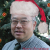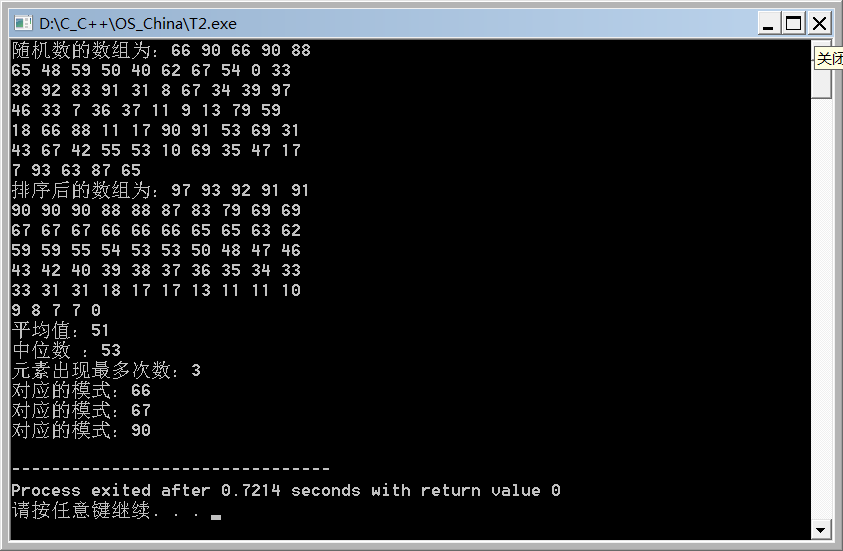# C++练习题

osc_94793021 发布于 2020/11/30 21:17

Activity 13
Statistical Analysis

Write a program that calculates the mean, median, and mode of a set of numbers. The mean is the sum of the numbers divided by the count of the numbers (ie: the average). The median is the number that sits in the middle of the data set (if there is an even number of data points, it is the average of the two middle values). The mode is the value that occurs most frequently in the data set. You can assume that the data set is already sorted.

0What Is the Mode?
The mode is the value that appears most frequently in a data set. A set of data may have one mode, more than one mode, or no mode at all.

``````#include <map>
#include <iostream>
#include <stdlib.h>
#include <time.h>

using namespace std;

void bubble_sort(int a[], int n){
int i,j,t;
int swap=1;
int count=1;

for(i=n-1; i>0 && swap==1;--i) {
swap=0; /* 用来监视此次循环，是否有相邻元素交换的情况发生。
* 		swap 为 0 (false)，表示没有交换情况发生，
*		为 1 (true)，表示有交换情况发生。
*/
for(j=0;j<i;++j)
if(a[j]<a[j+1]){
t=a[j];
a[j]=a[j+1];
a[j+1]=t;
swap=1;
/* swap 为 1，说明 有 相邻元素交换的情况发生。
*/
}
/* 如果 直到这次 for 循环结束，swap 保持 0，
*说明这次循环从头到尾都没有相邻元素交换得情况发生，
*这意味着排序完成，冒泡排序应当立刻结束。
*所以，for 循环的必要条件，除了 i > 0 之外，swap 必须为 1。
*/
}
};

void print(int a[], int n){
int i;
for (i=0;i<n ;i++){
cout<< a[i]<<" ";
if (i%10-4==0)
cout<<endl;
};
}

int main(){
const int N=60;
int data[N];
int i;

srand(time(NULL));
for (i=0;i<N;i++)
data[i]=rand()%100;

cout<<"随机数的数组为：";
print(data,N);
bubble_sort(data, N);
cout<<"\n排序后的数组为：";
print(data,N);

int sum = 0;
for (i=0;i<N;i++)
sum += data[i];
cout<<"\n平均值："<< sum/N << endl;

if (N%2==0)
cout <<"中位数 ：" << (data[N/2]+data[N/2-1]) /2 << endl ;
else
cout <<"中位数 ：" << data[N/2] << endl;

map<int, int> mapStudent;
for (i=0;i<N;i++)
mapStudent.insert(pair<int, int>(data[i], 0));

map<int, int>::iterator iter;
for(iter = mapStudent.begin(); iter != mapStudent.end(); iter++) {
int n=0;
for(i=0;i<N;i++)
if (iter->first == data[i])
iter->second++;
};

iter = mapStudent.begin();
int maxNumber= iter->second;
iter++;
for ( ; iter != mapStudent.end(); iter++)
if (maxNumber<iter->second)
maxNumber = iter->second;

if(maxNumber==1)
cout<<"没有模式值"<<endl;
else {

cout << "元素出现最多次数：" << maxNumber << endl;

for(iter = mapStudent.begin(); iter != mapStudent.end(); iter++)
if (maxNumber == iter->second)
cout<<"对应的模式："<< iter->first<<endl;
}
} ``````C

0
Y

0
C

反向代理服务器通常有两种模型，它可以作为内容服务器的替身，也可以作为内容服务器集群的负载均衡器。

1，作内容服务器的替身

如果您的内容服务器具有必须保持安全的敏感信息，如信用卡号数据库，可在防火墙外部设置一个代理服务器作为内容服务器的替身。当外部客户机尝试访问内容服务器时，会将其送到代理服务器。实际内容位于内容服务器上，在防火墙内部受到安全保护。代理服务器位于防火墙外部，在客户机看来就像是内容服务器。

当客户机向站点提出请求时，请求将转到代理服务器。然后，代理服务器通过防火墙中的特定通路，将客户机的请求发送到内容服务器。内容服务器再通过该通道将结果回传给代理服务器。代理服务器将检索到的信息发送给客户机，好像代理服务器就是实际的内容服务器。如果内容服务器返回错误消息，代理服务器会先行截取该消息并更改标头中列出的任何 URL，然后再将消息发送给客户机。如此可防止外部客户机获取内部内容服务器的重定向 URL。

这样，代理服务器就在安全数据库和可能的恶意攻击之间提供了又一道屏障。与有权访问整个数据库的情况相对比，就算是侥幸攻击成功，作恶者充其量也仅限于访问单个事务中所涉及的信息。未经授权的用户无法访问到真正的内容服务器，因为防火墙通路只允许代理服务器有权进行访问。

可以配置防火墙路由器，使其只允许特定端口上的特定服务器（在本例中为其所分配端口上的代理服务器）有权通过防火墙进行访问，而不允许其他任何机器进出。

2，作为内容服务器的负载均衡器

可以在一个组织内使用多个代理服务器来平衡各 Web 服务器间的网络负载。在此模型中，可以利用代理服务器的高速缓存特性，创建一个用于负载平衡的服务器池。此时，代理服务器可以位于防火墙的任意一侧。如果 Web 服务器每天都会接收大量的请求，则可以使用代理服务器分担 Web 服务器的负载并提高网络访问效率。

对于客户机发往真正服务器的请求，代理服务器起着中间调停者的作用。代理服务器会将所请求的文档存入高速缓存。如果有不止一个代理服务器，DNS 可以采用“循环复用法”选择其 IP 地址，随机地为请求选择路由。客户机每次都使用同一个 URL，但请求所采取的路由每次都可能经过不同的代理服务器。

可以使用多个代理服务器来处理对一个高用量内容服务器的请求，这样做的好处是内容服务器可以处理更高的负载，并且比其独自工作时更有效率。在初始启动期间，代理服务器首次从内容服务器检索文档，此后，对内容服务器的请求数会大大下降。